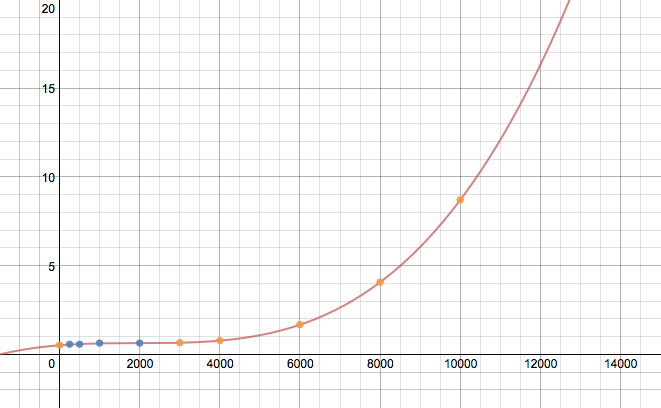# Extrapolate values in Pandas DataFrame

It’s very easy to interpolate NaN cells in a Pandas DataFrame:

```In : df
Out:
neg       neu       pos       avg
250    0.508475  0.527027  0.641292  0.558931
500         NaN       NaN       NaN       NaN
1000   0.650000  0.571429  0.653983  0.625137
2000        NaN       NaN       NaN       NaN
3000   0.619718  0.663158  0.665468  0.649448
4000        NaN       NaN       NaN       NaN
6000        NaN       NaN       NaN       NaN
8000        NaN       NaN       NaN       NaN
10000       NaN       NaN       NaN       NaN
20000       NaN       NaN       NaN       NaN
30000       NaN       NaN       NaN       NaN
50000       NaN       NaN       NaN       NaN

[12 rows x 4 columns]

In : df.interpolate(method='nearest', axis=0)
Out:
neg       neu       pos       avg
250    0.508475  0.527027  0.641292  0.558931
500    0.508475  0.527027  0.641292  0.558931
1000   0.650000  0.571429  0.653983  0.625137
2000   0.650000  0.571429  0.653983  0.625137
3000   0.619718  0.663158  0.665468  0.649448
4000        NaN       NaN       NaN       NaN
6000        NaN       NaN       NaN       NaN
8000        NaN       NaN       NaN       NaN
10000       NaN       NaN       NaN       NaN
20000       NaN       NaN       NaN       NaN
30000       NaN       NaN       NaN       NaN
50000       NaN       NaN       NaN       NaN

[12 rows x 4 columns]
```

I would also want it to extrapolate the NaN values that are outside of the interpolation scope, using the given method. How could I best do this?

# Extrapolating Pandas `DataFrame`s

`DataFrame`s maybe be extrapolated, however, there is not a simple method call within pandas and requires another library (e.g. scipy.optimize).

## Extrapolating

Extrapolating, in general, requires one to make certain assumptions about the data being extrapolated. One way is by curve fitting some general parameterized equation to the data to find parameter values that best describe the existing data, which is then used to calculate values that extend beyond the range of this data. The difficult and limiting issue with this approach is that some assumption about trend must be made when the parameterized equation is selected. This can be found thru trial and error with different equations to give the desired result or it can sometimes be inferred from the source of the data. The data provided in the question is really not large enough of a dataset to obtain a well fit curve; however, it is good enough for illustration.

The following is an example of extrapolating the `DataFrame` with a 3rd order polynomial

f(x) = a x3 + b x2 + c x + d (Eq. 1)

This generic function (`func()`) is curve fit onto each column to obtain unique column specific parameters (i.e. a, b, c, d). Then these parameterized equations are used to extrapolate the data in each column for all the indexes with `NaN`s.

```import pandas as pd
from cStringIO import StringIO
from scipy.optimize import curve_fit

df = pd.read_table(StringIO('''
neg       neu       pos       avg
0           NaN       NaN       NaN       NaN
250    0.508475  0.527027  0.641292  0.558931
500         NaN       NaN       NaN       NaN
1000   0.650000  0.571429  0.653983  0.625137
2000        NaN       NaN       NaN       NaN
3000   0.619718  0.663158  0.665468  0.649448
4000        NaN       NaN       NaN       NaN
6000        NaN       NaN       NaN       NaN
8000        NaN       NaN       NaN       NaN
10000       NaN       NaN       NaN       NaN
20000       NaN       NaN       NaN       NaN
30000       NaN       NaN       NaN       NaN
50000       NaN       NaN       NaN       NaN'''), sep='s+')

# Do the original interpolation
df.interpolate(method='nearest', xis=0, inplace=True)

# Display result
print ('Interpolated data:')
print (df)
print ()

# Function to curve fit to the data
def func(x, a, b, c, d):
return a * (x ** 3) + b * (x ** 2) + c * x + d

# Initial parameter guess, just to kick off the optimization
guess = (0.5, 0.5, 0.5, 0.5)

# Create copy of data to remove NaNs for curve fitting
fit_df = df.dropna()

# Place to store function parameters for each column
col_params = {}

# Curve fit each column
for col in fit_df.columns:
# Get x & y
x = fit_df.index.astype(float).values
y = fit_df[col].values
# Curve fit column and get curve parameters
params = curve_fit(func, x, y, guess)
# Store optimized parameters
col_params[col] = params

# Extrapolate each column
for col in df.columns:
# Get the index values for NaNs in the column
x = df[pd.isnull(df[col])].index.astype(float).values
# Extrapolate those points with the fitted function
df[col][x] = func(x, *col_params[col])

# Display result
print ('Extrapolated data:')
print (df)
print ()

print ('Data was extrapolated with these column functions:')
for col in col_params:
print ('f_{}(x) = {:0.3e} x^3 + {:0.3e} x^2 + {:0.4f} x + {:0.4f}'.format(col, *col_params[col]))
```

## Extrapolating Results

```Interpolated data:
neg       neu       pos       avg
0           NaN       NaN       NaN       NaN
250    0.508475  0.527027  0.641292  0.558931
500    0.508475  0.527027  0.641292  0.558931
1000   0.650000  0.571429  0.653983  0.625137
2000   0.650000  0.571429  0.653983  0.625137
3000   0.619718  0.663158  0.665468  0.649448
4000        NaN       NaN       NaN       NaN
6000        NaN       NaN       NaN       NaN
8000        NaN       NaN       NaN       NaN
10000       NaN       NaN       NaN       NaN
20000       NaN       NaN       NaN       NaN
30000       NaN       NaN       NaN       NaN
50000       NaN       NaN       NaN       NaN

Extrapolated data:
neg          neu         pos          avg
0         0.411206     0.486983    0.631233     0.509807
250       0.508475     0.527027    0.641292     0.558931
500       0.508475     0.527027    0.641292     0.558931
1000      0.650000     0.571429    0.653983     0.625137
2000      0.650000     0.571429    0.653983     0.625137
3000      0.619718     0.663158    0.665468     0.649448
4000      0.621036     0.969232    0.708464     0.766245
6000      1.197762     2.799529    0.991552     1.662954
8000      3.281869     7.191776    1.702860     4.058855
10000     7.767992    15.272849    3.041316     8.694096
20000    97.540944   150.451269   26.103320    91.365599
30000   381.559069   546.881749   94.683310   341.042883
50000  1979.646859  2686.936912  467.861511  1711.489069

Data was extrapolated with these column functions:
f_neg(x) = 1.864e-11 x^3 + -1.471e-07 x^2 + 0.0003 x + 0.4112
f_neu(x) = 2.348e-11 x^3 + -1.023e-07 x^2 + 0.0002 x + 0.4870
f_avg(x) = 1.542e-11 x^3 + -9.016e-08 x^2 + 0.0002 x + 0.5098
f_pos(x) = 4.144e-12 x^3 + -2.107e-08 x^2 + 0.0000 x + 0.6312
```

## Plot for `avg` columnWithout a larger dataset or knowing the source of the data, this result maybe completely wrong, but should exemplify the process to extrapolate a `DataFrame`. The assumed equation in `func()` would probably need to be played with to get the correct extrapolation. Also, no attempt to make the code efficient was made.

Update:

If your index is non-numeric, like a `DatetimeIndex`, see this answer for how to extrapolate them.